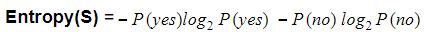Search

# Decision Tree Classification in Python : Everything you need to know

## What is Decision Tree?

A decision tree is a decision support tool that uses a tree-like graph or model of decisions and their possible consequences, including chance event outcomes, resource costs, and utility. It is one way to display an algorithm that only contains conditional control statements.

Decision Trees (DTs) are a non-parametric supervised learning method used for both classification and regression. Decision trees learn from data to approximate a sine curve with a set of if-then-else decision rules. The deeper the tree, the more complex the decision rules, and the fitter the model. The decision tree builds classification or regression models in the form of a tree structure, hence called CART (Classification and Regression Trees). It breaks down a data set into smaller and smaller subsets building along an associated decision tree at the same time. The final result is a tree with decision nodes and leaf nodes. A decision node has two or more branches. The leaf node represents a classification or decision. The topmost decision node in a tree which corresponds to the best predictor called the root node. Decision trees can handle both categorical and numerical data.

## When is Decision Tree Used?

1. When the user has and objective and he is trying to achieve max profit, optimized cost, etc.

2. When there are several courses of action like the menu system in an ATM machine, Customer Support calling menu, etc.

3. Uncertainty concerning which outcome will actually happen.

## How to Make a Decision Tree?

Step 1

Calculate the entropy of the target.

Step 2

The dataset is then split into different attributes. The entropy for each branch is calculated. Then it is added proportionally, to get total entropy for the split. The resulting entropy is subtracted from the entropy before the split. The result is the Information Gain or decrease in entropy.

Step 3

Choose attribute with the largest information gain as the decision node, divide the dataset by its branches and repeat the same process on every branch.

## EntropyWhere,

• S is the total sample space,

• P(yes) is the probability of yes

If number of yes = nunmber of no i.e. P(S) = 0.5

• Entropy(S) = 1 When P(yes) = P(no) = 0.5 i.e. YES +NO = Total Sample(S) = 1

If it contains all yes or all no i.e. P(S) = 1 or 0

Entropy(S) = 0 When P(yes) = 1 i.e. YES = Total Sample(S) E(S) = 1 log 1

E(S) = 0

## Information Gain

• Measure the reduction in entropy

• Decides which attribute should be selected as a decision node.

If S is our total collection,

Information Gain = Entropy(S) - [(Weighted Avg) x Entropy(each feature)]

## We will use the following libraries.

1. Python Pandas

2. Python Numpy

3. Python Scikit Learn

4. Python MatPlotLib

We will use the BankNoteAuthentication dataset.

```import pandas as pd
import numpy as np
import matplotlib.pyplot as plt
%matplotlib inline

bankdata```### Feature Selection

Here, you need to divide given columns into two types of variables dependent(or target variable) and independent variable(or feature variables).

```feature_cols = ['variance','skewness','curtosis','entropy']
#split dataset in features and target variable
X = pima[feature_cols] # Features
y = pima['class'] # Target variable```

### Splitting Data

To understand model performance, dividing the dataset into a training set and a test set is a good strategy.

Let's split the dataset by using function train_test_split(). You need to pass 3 parameters features, target, and test_set size.

```# Split dataset into training set and test set
X_train, X_test, y_train, y_test = train_test_split(X, y, test_size=0.3, random_state=1) # 70% training and 30% test```

### Building Decision Tree Model

Let's create a Decision Tree Model using Scikit-learn.

```# Create Decision Tree classifer object
clf = DecisionTreeClassifier()
# Train Decision Tree Classifer
clf = clf.fit(X_train,y_train)
#Predict the response for test dataset
y_pred = clf.predict(X_test)```

### Evaluating Model

Let's estimate, how accurately the classifier or model can predict the type of cultivars.

Accuracy can be computed by comparing actual test set values and predicted values.

```# Model Accuracy, how often is the classifier correct?
print("Accuracy:",metrics.accuracy_score(y_test, y_pred))```

OUTPUT: Accuracy: 0.9878640776699029

### Confusion matrix

A confusion matrix is a summary of prediction results on a classification problem. The number of correct and incorrect predictions are summarized with count values and broken down by each class. This is the key to the confusion matrix. The confusion matrix shows the ways in which your classification model is confused when it makes predictions. It gives us insight not only into the errors being made by a classifier but more importantly the types of errors that are being made.

```cm = confusion_matrix(y_test, y_pred)
cm ```

OUTPUT: array([[231, 4], [ 1, 176]])